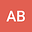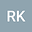Existence of solution to a Dirichlet elliptic problem on the Sierpi´nski gasket
••• Rasmita Kar
NIT Rourkela

Corresponding Author:ons.arun93@gmail.com

Author ProfileRasmita Kar
NIT Rourkela
Author Profile## Abstract

We study the existence of a weak (strong) solution of the nonlinear elliptic problem \begin{equation*} \begin{gathered} -\Delta u- \lambda ug_1 +h(u)g_2=f \quad\text{in } V\setminus V_0\\ u=0 \quad\text{on } V_0, \end{gathered}\end{equation*} where \small{$V$} is the Sierpi\’nski gasket in \small{$\mathbb{R}^{N-1}(N\geq 2)$, $V_0$} is its boundary (consisting of its \small{$N$} corners) and $\lambda$ is a real parameter. Here, $f,g_1,g_2:V\to\mathbb{R}$, $h:\mathbb{R}\to\mathbb{R}$ are functions satisfying suitable hypotheses.